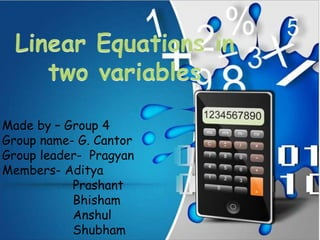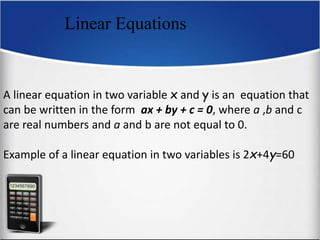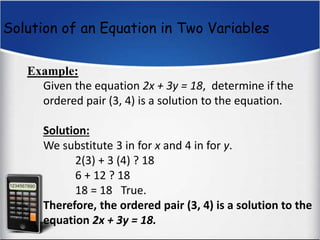Seu SlideShare está sendo baixado. ×

# Linear equations in two variables- By- Pragyan

Anúncio
Anúncio
Anúncio
Anúncio
Anúncio
Anúncio
Anúncio
Anúncio
Anúncio
Anúncio
AnúncioCarregando em…3
×

1 de 18 Anúncio

# Linear equations in two variables- By- Pragyan

This is a power point presentation on linear equations in two variables for class 10th. I have spent 3 hours on making this and all the equations you will see are written by me.

This is a power point presentation on linear equations in two variables for class 10th. I have spent 3 hours on making this and all the equations you will see are written by me.

Anúncio
Anúncio

### Linear equations in two variables- By- Pragyan

1. 1. Made by – Group 4 Group name- G. Cantor Group leader- Pragyan Members- Aditya Prashant Bhisham Anshul Shubham
2. 2. Linear Equations A linear equation in two variable x and y is an equation that can be written in the form ax + by + c = 0, where a ,b and c are real numbers and a and b are not equal to 0. Example of a linear equation in two variables is 2x+4y=60
3. 3. Solution of an Equation in Two Variables Example: Given the equation 2x + 3y = 18, determine if the ordered pair (3, 4) is a solution to the equation. Solution: We substitute 3 in for x and 4 in for y. 2(3) + 3 (4) ? 18 6 + 12 ? 18 18 = 18 True. Therefore, the ordered pair (3, 4) is a solution to the equation 2x + 3y = 18.
4. 4. The Rectangular Coordinate System In the rectangular coordinate system, the horizontal number line is the x-axis. The vertical number line is the y-axis. The point of intersection of these axes is their zero points, called the origin. The axes divide the plane into 4 quarters, called quadrants.
5. 5. CARTESIAN PLANE Quadrant II ( - ,+) Quadrant I (+,+) Quadrant IV (+, - ) Quadrant III ( - , - ) y-axis x- axis
6. 6. Plotting Points on a Graph Paper EXAMPLE Plot the points (3,2) and (-2,-4). SOLUTION
7. 7. Solution for a linear equation in two variables Let ax + by +c = O , where a ,b , c are real numbers such that a and b ≠ O. Then, any pair of values of x and y which satisfies the equation ax + by +c = O, is called a solution of it.
8. 8. Graphical Method Example Solve the following system of linear equations: Since we are seeking out the point of intersection, we may graph the equations: We see here that the lines intersect each other at the point x = 2, y = 8. This is our solution and we may refer to it as a graphic solution to the task.
9. 9. Substitution method The method of solving "by substitution" works by solving one of the equations (you choose which one) for one of the variables (you choose which one), and then plugging this back into the other equation, "substituting" for the chosen variable and solving for the other. Then you back-solve for the first variable.
10. 10. For example if the equations are 푥 − 2푦 = 10…… (i) and 2푥 + 푦 = 30………. (ii) From (i) we get 푥 = 10 + 2푦 So now we put the value of x in equation (ii)…. 2 10 + 2푦 + 푦 = 30 Or, 20 + 5푦 = 30 Or, 푦 = 10 5 Or, 푦 = 2 Putting the value of y in equation (i) i.e. 푥 − 2 2 = 10 or, 푥 = 10 + 4 표푟, 푥 = 14
11. 11. Elimination In the eliMmineattiohn moedthod we eliminate either of the variables to solve the equation. We first make the coefficients of the variable equal in both the equations by multiplying the whole equation by an integer except zero. Then we eliminate the variable and solve the equation for the value of another variable.
12. 12. For example if the equations are 푥 − 2푦 = 10…… (i) and 2푥 + 푦 = 30………. (ii) First we make the coefficient of x in equation (i) equal to that of equation (ii). So, we multiply the whole equation by 2 we get, 2푥 − 4푦 = 20 … … … (푖푖푖) Now we can either subtract (ii) by (iii) or vice versa. So, subtracting (iii) by (ii) we get, 2푥 + 푦 − 2푥 − 4푦 = 30 − 20 푂푟, 2푥 + 푦 − 2푥 + 4푦 = 10 푂푟, 5푦 = 10 푖. 푒. 푦 = 10 5 푖. 푒. 푦 = 2 Now we put the value of y in equation (iii) we get, 2푥 − 4 2 = 20 푖. 푒. 2푥 = 20 + 8 푖. 푒. 2푥 = 28 표푟, 푥 = 28 2 표푟, 푥 = 14
13. 13. Cross multiplication method Let’s consider the general form of a pair of linear equations a1x + b1y + c1 = 0 , and a2x + b2y + c2 = 0. When a1 divided by a2 is not equal to b1 divided by b2, the pair of linear equations will have a unique solution. To solve this pair of equations for x and y using cross-multiplication, we’ll arrange the variables x and y and their coefficients a1, a2, b1 and b2, and the constants c1 and c2 as shown
14. 14. For example if the equations are 푥 − 2푦 = 10…… (i) and 2푥 + 푦 = 30………. (ii) First we write it in this form as 푥 − 2푦 − 10 = 0 푎푛푑 2푥 + 푦 − 30 = 0 According to the theory 푥 −2 − 10 1 − 30 = 푦 −10 1 −30 2 = 1 1 − 2 2 1 Cross multiplying we get, 푥 −10 − 60 = 푦 −30 − −20 = 1 −4 − 1 푖푒. 푥 −70 = 푦 −10 = 1 −5 푥 −70 = 1 −5 푖푒. −5푥 = −70 푖푒. 푥 = − 70 −5 표푟, 푥 = 14 푦 −10 = 1 −5 푖푒. −5푦 = −10 푖푒. 푦 = − 10 −5 푖푒. 푦 = 2
15. 15. Equations reducible to a pair of linear equations in two variables Q. Solve the pair of equations 2 푥 + 3 푦 = 13 5 푥 − 4 푦 = −2 Sol. Let us write the equations as 2 1 푥 + 3 1 푦 = 13 5 1 푥 − 4 1 푦 = −2
16. 16. We let 1 푥 = 푝 푎푛푑 1 푦 = 푞 Thus the equation becomes, 2푝 + 3푞 = 13 푎푛푑 5푝 − 4푞 = −2 Now you can use any method to get p=2 and q=3 Substituting the values of p and q to get, 1 푥 = 2 푖푒. , 푥 = 1 2 푎푛푑 1 푦 = 3 푖푒. , 푦 = 1 3